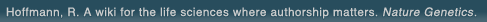# The world's first wiki where authorship really matters (Nature Genetics, 2008). Due credit and reputation for authors. Imagine a global collaborative knowledge base for original thoughts. Search thousands of articles and collaborate with scientists around the globe.

wikigene or wiki gene protein drug chemical gene disease author authorship tracking collaborative publishing evolutionary knowledge reputation system wiki2.0 global collaboration genes proteins drugs chemicals diseases compound
Hoffmann, R. A wiki for the life sciences where authorship matters. Nature Genetics (2008)# Relationship between run times to exhaustion at 90, 100, 120, and 140% of vVO2max and velocity expressed relatively to critical velocity and maximal velocity.

The aim of the present study was to explain the inter-individual variability in running time to exhaustion (tlim) when running speed was expressed as a percentage of the velocity, associated with maximal oxygen uptake (vVO2max). Indeed for the same percentage of vVO2max the anaerobic contribution to energy supply is different and could be dependent on the critical velocity (Cv) and also on the maximal running velocity (vmax). Ten subjects ran four tlim at 90, 100, 120, and 140% of vVO2max; mean and standard deviation for tlim were 839 +/- 236 s, 357 +/- 110 s, 122 +/- 27 s, and 65 +/- 17s, respectively. Each velocity was then expressed 1) as a percentage of the difference between vVO2max and Cv (%AeSR); 2) as a percentage of the difference between vmax and Cv (% MSR); 3) as a percentage of the difference between vmax and vVO2max (%AnSR). Highest correlations were found between tlim90 and tlim100 and velocity expressed as % MSR (r = -0.82, p < 0.01 and r = -0.75, p < 0.01), and between tlim120 and tlim140 and velocity expressed as %AnSR (r = -0.83, p < 0.01 and r = -0.94, p < 0.001). These results show that the same intensity relative to aerobic contribution did not represent the same absolute intensity for all and could partly explain variability in tlim. Therefore expressing intensity as a percentage of MSR for sub-maximal and maximal velocities and as a percentage of AnSR for supra-maximal velocities allows individual differences in anaerobic work capacity to be taken into account and running times to exhaustion to be predicted accurately.